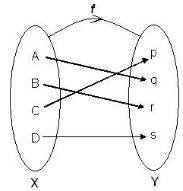# Test: Problems On Functions

## 10 Questions MCQ Test Mathematics (Maths) Class 12 | Test: Problems On Functions

Description
This mock test of Test: Problems On Functions for JEE helps you for every JEE entrance exam. This contains 10 Multiple Choice Questions for JEE Test: Problems On Functions (mcq) to study with solutions a complete question bank. The solved questions answers in this Test: Problems On Functions quiz give you a good mix of easy questions and tough questions. JEE students definitely take this Test: Problems On Functions exercise for a better result in the exam. You can find other Test: Problems On Functions extra questions, long questions & short questions for JEE on EduRev as well by searching above.
QUESTION: 1

### Let A be the set of all 25 students of Class X in a school. Let f : A → N be function defined by f (x) = roll number of the student x. Then f is:​

Solution:

f(x) = Roll number of student x
f(x1) = Roll number of student x1
f(x2) = Roll number of student x2
Putting f(x1) = f(x2)
Roll number of student x1 = Roll number of student x2
Since no two student of a class have same roll number.
Therefore, x1 ≠ x2
So, f is one-one.

QUESTION: 2

### A function f: A x B → B x A defined by f (a, b) = (b, a) on two sets A and B. The function is:​

Solution:

f:A×B→B×A is defined as f(a,b)=(b,a).
Let (a1,b1),(a2,b2)∈A×B such that f(a1,b1)=f(a2,b2).
⇒(b1,a1)=(b2,a2)
⇒(b1=b2) and (a1=a2)
⇒(a1,b1)=(a2,b2)
∴f is one-one.
Now, let (b,a)∈B×A be any element.
Then, there exists (a,b)∈A×B such that
f(a,b)=(b,a). [By definition of f]
∴ f is onto.

QUESTION: 3

### Let  f : N→ R - {0} defined as f(x) = 1/x where x ∈ N is not an onto function. Which one of the following sets should be replaced by N such that the function f will become onto?​ (where R0 = R - {0})

Solution:
QUESTION: 4

The function f isSolution:

The function is bijective (one-to-one and onto or one-to-one correspondence) if each element of the codomain is mapped to by exactly one element of the domain. (That is, the function is both injective and surjective.) A bijective function is a bijection.

QUESTION: 5

A function f: R → R  is defined by f(x) = [x+1], where [x] the greatest integer function, is:​

Solution:

Explanation : f: R → R is given by,

f(x) = [x+1]

It is seen that f(1.2) = [1.2] = 1, f(1.9) = [1.9] = 1.

∴ f(1.2) = f(1.9), but 1.2 ≠ 1.9.

∴ f is not one-one.

Now, consider 0.7 ∈ R.

It is known that f(x) = [x] is always an integer. Thus, there does not exist any element x ∈ R such that f(x) = 0.7.

∴ f is not onto.

Hence, the greatest integer function is neither one-one nor onto.

QUESTION: 6

How many onto functions from set A to set A can be formed for the set A = {1, 2, 3, 4, 5, ……n}?​

Solution:

Taking set {1,2,3}
Since f is onto,all elements of{1,2,3} have unique pre-image.
Total no. of one-one function = 3×2×1=6
Eg:- Since f is onto,all elements of {1,2,3} have unique pre-image.
total no. of onto functions = n×n−1×n−2×2×1
= n!

QUESTION: 7

Let A = {1, 2, 3}. f: A → A Then complete the function f such that it one-one and onto: f = {(1, 2), (2,1) ______}

Solution:
QUESTION: 8

If a relation f: X → Y is a function, then for g: Y → X to be a function, function f need to be​

Solution:
QUESTION: 9

For which one of these mapping the function f(x) = x2 will be one-one?​

Solution:

consider ‘f’ is a function whose domain is set A. The function is said to be injective if for all x and y in A,
Whenever f(x)=f(y), then x=y
And equivalently, if x ≠ y, then f(x) ≠ f(y)
Formally, it is stated as, if f(x) = f(y)  implies x=y, then f is one-to-one mapped, or f is 1-1.

QUESTION: 10

Let f and g be the function from the set of integers to itself, defined by f(x) = 2x + 1 and g(x) = 3x + 4. Then the composition of f and g is ____________

Solution:

The composition of f and g is given by f (g(x)) which is equal to 2 (3x + 4) + 1.